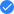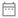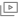Back to Plus

# Complete Course on Engineering Mathematics5k followers

In this course, Sanjay Yadav will provide in-depth knowledge of Engineering Mathematics. The course includes 57 sessions of 90 minutes each. All the important topics such as Introduction to Engineering Mathematics, Limit, and Continuity, etc will be discussed in detail and would be helpful for aspirants preparing for the GATE exam. Learners at any stage of their preparations will be benefited by the course. The course will be covered in Hindi and notes will be provided in English.
Course schedule
Aug
17
Introduction to Engineering Mathematics
Lesson 14:00 AM
Aug
18
Introduction to Matrix and Properties
Lesson 24:00 AM
Aug
19
System of Linear Equation and Rank of Matrix
Lesson 34:00 AM
Aug
20
Doubt Clearing Session
Lesson 44:00 AM
Aug
21
Eigen Value and Eigen Vectors
Lesson 54:00 AM
Aug
22
Limit and Continuity
Lesson 64:00 AM
Aug
23
Concept of Differentiability
Lesson 74:00 AM
Aug
23
Weekly Quiz
Lesson 811:30 AM
Aug
24
Doubt Clearing Session
Lesson 94:00 AM
Aug
25
Maxima and Minima
Lesson 104:00 AM
Aug
26
Mean Value Theorem
Lesson 114:00 AM
Aug
27
Concept of Partial Derivatives
Lesson 124:00 AM
Aug
28
Doubt Clearing Session
Lesson 134:00 AM
Aug
29
Concept of Definite and Indefinite Integral
Lesson 144:00 AM
Aug
30
Multiple Integral
Lesson 154:00 AM
Aug
30
Weekly Quiz
Lesson 1611:30 AM
Sep
01
Area Under the Curve - II
Lesson 1712:30 PM
Sep
01
Doubt Clearing Session
Lesson 182:30 PM
Sep
02
Improper Integral
Lesson 194:00 AM
Sep
03
Introduction to Vector Calculus and Gradient
Lesson 204:00 AM
Sep
04
Directional Derivatives
Lesson 214:00 AM
Sep
06
Concept of Divergence and Curl
Lesson 224:00 AM
Sep
06
Weekly Quiz
Lesson 2311:30 AM
Sep
07
Integral Calculus : Line Integral
Lesson 244:00 AM
Sep
08
Integral Calculus : Surface Integral & Volume Integral
Lesson 254:00 AM
Sep
09
Green, Gauss and Stokes Theorem
Lesson 264:00 AM
Sep
10
Doubt Clearing Session
Lesson 274:00 AM
Sep
11
Numerical Method: Solution of Non Algebraic Equation
Lesson 284:00 AM
Sep
12
Integration by Trapezoidal and Simpson's Rule
Lesson 294:00 AM
Sep
13
Single and Multi Step Method for Differential Equation
Lesson 304:00 AM
Sep
13
Weekly Quiz
Lesson 3111:30 AM
Sep
14
Doubt Clearing Session
Lesson 324:00 AM
Sep
15
Introduction to Complex Variable
Lesson 334:00 AM
Sep
16
Analytic Function & Cauchy Riemann Equation
Lesson 344:00 AM
Sep
20
Weekly Quiz
Lesson 3511:30 AM
Sep
21
Introduction to Differential Equation
Lesson 365:30 PM
Sep
22
Doubt Clearing Session
Lesson 374:00 AM
Sep
22
Solution of a Differential Equation
Lesson 385:30 PM
Sep
24
Linear, Bernoulli's and Exact Differential Equation
Lesson 394:00 AM
Sep
25
Higher Order Differential Equation
Lesson 404:00 AM
Sep
26
Doubt Clearing Session
Lesson 414:00 AM
Sep
27
Initial and Boundary Value Problem
Lesson 424:00 AM
Sep
27
Weekly Quiz
Lesson 4311:30 AM
Sep
28
Partial Differential Equation
Lesson 444:00 AM
Sep
28
Harmonic Conjugate
Lesson 455:30 PM
Sep
29
Introduction to Probability
Lesson 464:00 AM
Sep
29
Doubt Clearing Session
Lesson 475:30 PM
Sep
30
Doubt Clearing Session
Lesson 484:00 AM
Oct
01
Baye's Theorem and GATE PYQs
Lesson 494:00 AM
Oct
02
Binomial, Poisson and Normal Distribution
Lesson 504:00 AM
Oct
03
Statistics and Regression Analysis
Lesson 514:00 AM
Oct
04
Doubt Clearing Session
Lesson 524:00 AM
Oct
04
Weekly Quiz
Lesson 5311:30 AM
Oct
05
Laplace Transform - Part I
Lesson 544:00 AM
Oct
05
Integral Theorem and Residues
Lesson 555:30 PM
Oct
06
Laplace Transform - Part II
Lesson 564:00 AM
Oct
06
Taylors and Laurent Series
Lesson 575:30 PM
Oct
07
Z- Transform
Lesson 584:00 AM
Oct
08
Doubt Clearing Session
Lesson 594:00 AM
Oct
09
Fourier Transformation - Part I
Lesson 604:00 AM
Oct
10
Fourier Transformation - Part II
Lesson 614:00 AM
Oct
10
Weekly Quiz
Lesson 6211:30 AM
Oct
11
Revision Class
Lesson 634:00 AM
Oct
12
Doubt Clearing Session
Lesson 644:00 AM
Oct
12
Doubt Clearing Session
Lesson 6512:30 PM
Oct
13
Final Doubt Clearing Session
Lesson 664:00 AM
Oct
13
Mega Quiz
Lesson 6711:30 AMCourse will be taught in Hindi17th August to 13th October 201958 lessons, 9 quizzes